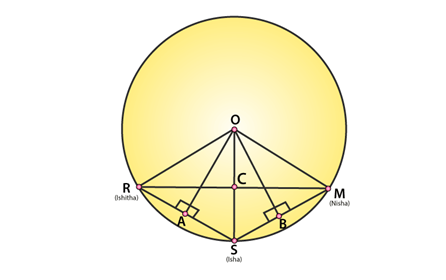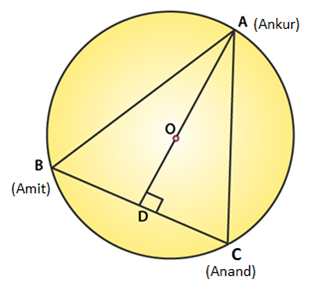• +91 9971497814
• info@interviewmaterial.com

# RD Chapter 16- Circles Ex-16.3 Interview Questions Answers

### Related Subjects

Question 1 : Three girls Ishita, Isha and Nisha are playing a game by standing on a circle of radius 20 m drawn in a park. Ishita throws a ball to Isha, Isha to Nisha and Nisha to Ishita. If the distance between Ishita and Isha and between Isha and Nisha is 24 m each, what is the distance between Ishita and Nisha.

Answer 1 : Let R, S and M be the position of Ishita, Isha and Nisha respectively.Since OA is a perpendicular bisector on RS, so AR = AS = 24/2 =12 cm

Radii of circle = OR = OS = OM = 20 cm (Given)

In ΔOAR:

By Pythagoras theorem,

OA2+AR2=OR2

OA2+122=202

OA= 400 – 144 =256

Or OA = 16 m …(1)

From figure, OABC is a kite since OA = OC and AB = BC. We knowthat, diagonals of a kite are perpendicular and the diagonal common to both theisosceles triangles is bisected by another diagonal.

So in ΔRSM, RCS = 900 and RC = CM …(2)

Now, Area of ΔORS = Area of ΔORS

=>1/2×OA×RS = 1/2 x RC x OS

=> OA ×RS = RC x OS

=> 16 x 24 = RC x 20

=> RC = 19.2

Since RC = CM (from (2), we have

RM = 2(19.2) = 38.4

So, the distance between Ishita and Nisha is 38.4 m.

Question 2 : A circular park of radius 40 m is situated in a colony. Three boys Ankur, Amit and Anand are sitting at equal distance on its boundary each having a toy telephone in his hands to talk to each other. Find the length of the string of each phone.

Answer 2 :Since, AB = BC = CA. So, ABC is an equilateral triangle

Radius = OA = 40 m (Given)

We know, medians of equilateral triangle pass through thecircumcentre and intersect each other at the ratio 2 : 1.

Here AD is the median of equilateral triangle ABC, we can write:

OA/OD = 2/1

or 40/OD = 2/1

or OD = 20 m

Therefore, AD = OA + OD = (40 + 20) m = 60 m

By Pythagoras theorem,

AC2 = 602 + (AC/2) 2

AC= 3600 + AC2 / 4

3/4 AC= 3600

AC= 4800

or AC = 40√3 m

Therefore, length of string of each phone will be 40√3 m.

Todays Deals### RD Chapter 16- Circles Ex-16.3 Contributorskrishan

Name:
Email:

# Latest News# 9000 interview questions in different categories